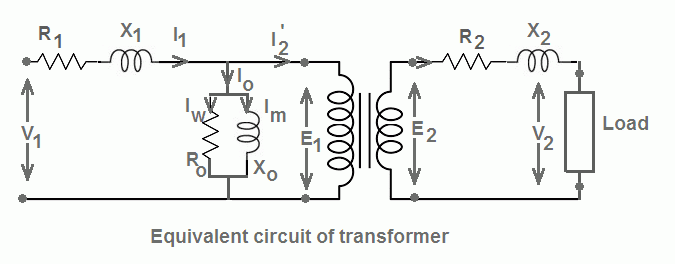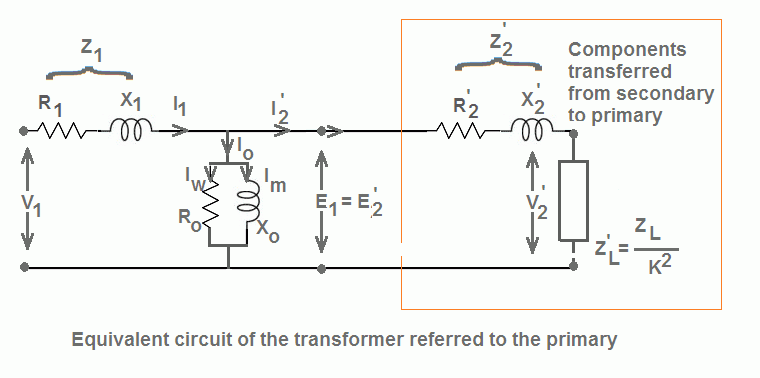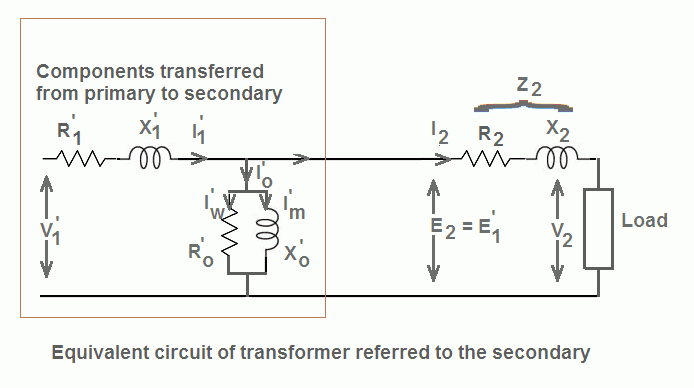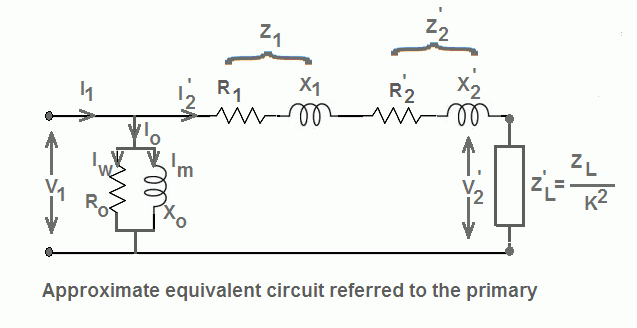# Equivalent Circuit of Transformer

The equivalent circuit of transformer is shown in the figure.The no-load primary current Io has two components, namely Im and Iw.

Where Im = magnetizing component = Io sin φo
and Iw = core-loss component = Io cos φo.

• Iw supplies for the no-load losses and is assumed to flow through the no-load resistance which is also known as core-loss resistance (Ro).
•

• The magnetizing component, Im is assumed to be flowing through a reactance which is known as magnetizing reactance, Xo.
•

• The parallel combination of Ro and Xo is also known as the exciting circuit.
• From the equivalent circuit of transformer,

Ro = V1/Iw and Xo = V1/Im.

## Primary Components

• The resistance R1 and reactance X1 correspond to the winding resistance (DC resistance) and leakage reactance of the primary winding.
•

• The total current I1 on the primary side is equal to the phasor sum of Io and I2’.
•

• I2’ = KI2 is the additional primary current which flows due to the load connected on the secondary side of the transformer.

## Secondary Components

• The resistance R2 and reactance X2 correspond to the winding resistance and leakage reactance of the secondary winding.
•

• Load impedance ZL can be resistive, inductive or capacitive.
•

• The equivalent circuit of single phase transformer is further simplified by transferring all the quantities to either primary or secondary side.
•

• This is done in order to make the calculations easy.

## Equivalent Circuit of Transformer Referred to PrimaryAll the components on the secondary side of the transformer are transferred to the primary side as shown in the figure.

• R2’, X2’ and ZL’ are the values of R2, X2 and ZL referred to primary respectively.
•

• The values of these components are obtained as follows:
•
R2’ = R2/K2 , X2’ = X2/K2 and ZL’ = ZL/K2

where K = N2/N1 (transformation ratio).

• The current I2 and voltage E2 are also transferred to the primary side as I2’ and E2’ respectively. The expressions for I2’ and E2’ are as follows:
•
E2’ = E2/K  and  I2’ = KI2

## Equivalent Circuit of Transformer Referred to SecondaryThe equivalent circuit of transformer referred to the secondary side is shown in the figure.

• Components R1’, X1’, Ro’ and Xo’ are the primary components referred to secondary. The expressions for these components are as follows:
•
R1’ = K2R1 ,  X1’ = K2X1

Ro’ = K2Ro ,  Xo’ = K2Xo

• The primary voltages and currents also get transferred to the secondary side as I1’, V1’, Io’, E1’ respectively and are given by:
•
I1’ = I1/K , E1’ = KE1 , Io’ = Io/K

where K = N2/N1 (transformation ratio).

# Approximate Equivalent Circuit of TransformerAn approximate equivalent circuit is one which is obtained by shifting the exciting circuit to the left of R1 and X1 as shown in the figure.

Although this shifting creates an error in the voltage drop across R1 and X1 yet it greatly simplifies the calculation work and gives much simplified equivalent circuit.

Transformer | All Posts

© https://yourelectricalguide.com/ equivalent circuit of transformer.